Ex 13.4

Chapter 13 Class 10 Surface Areas and Volumes
Serial order wise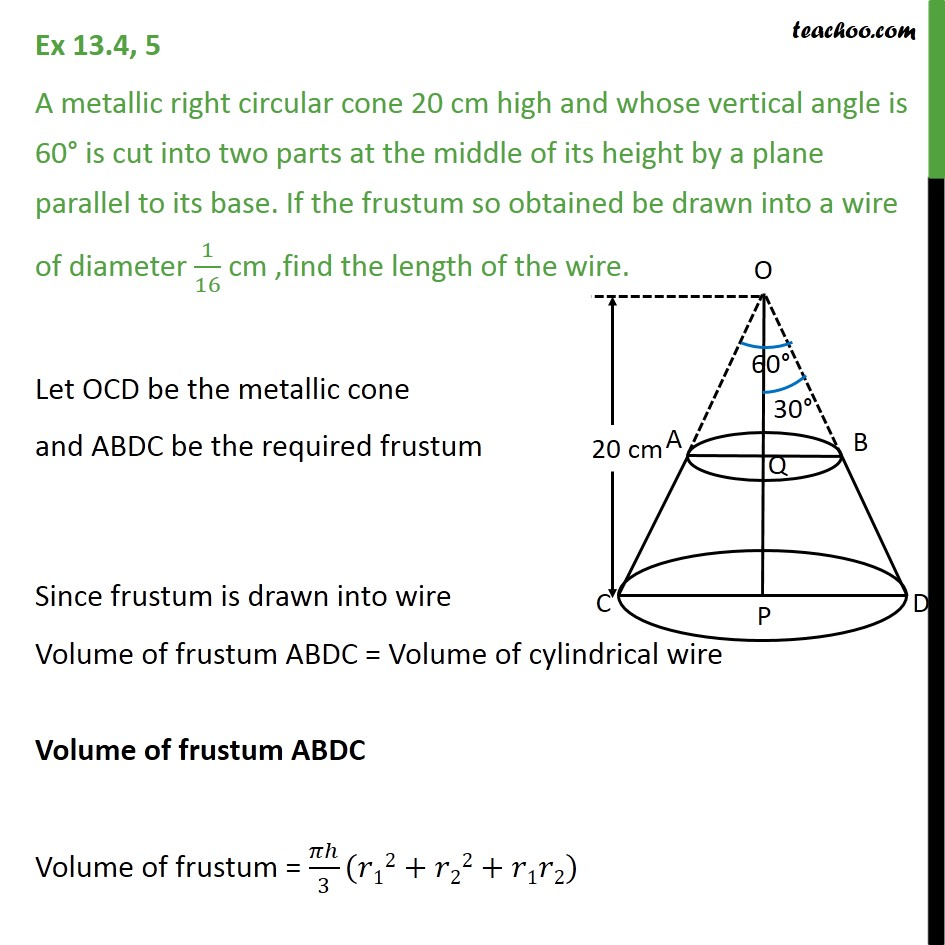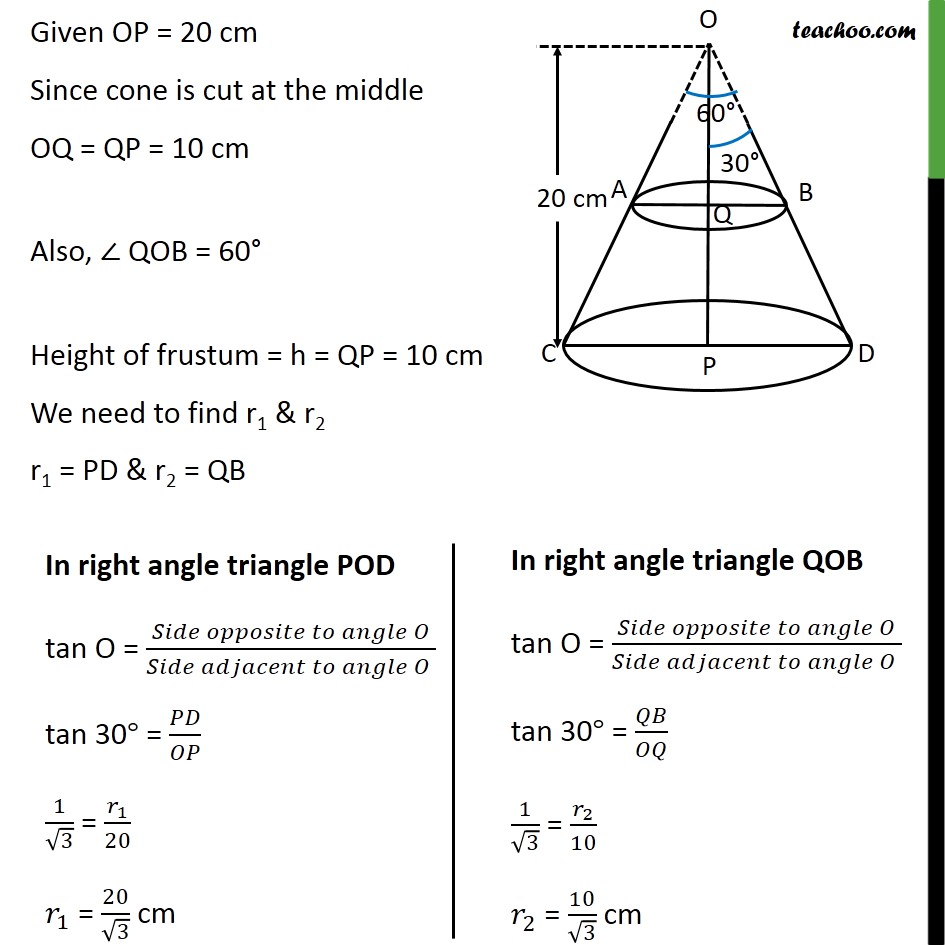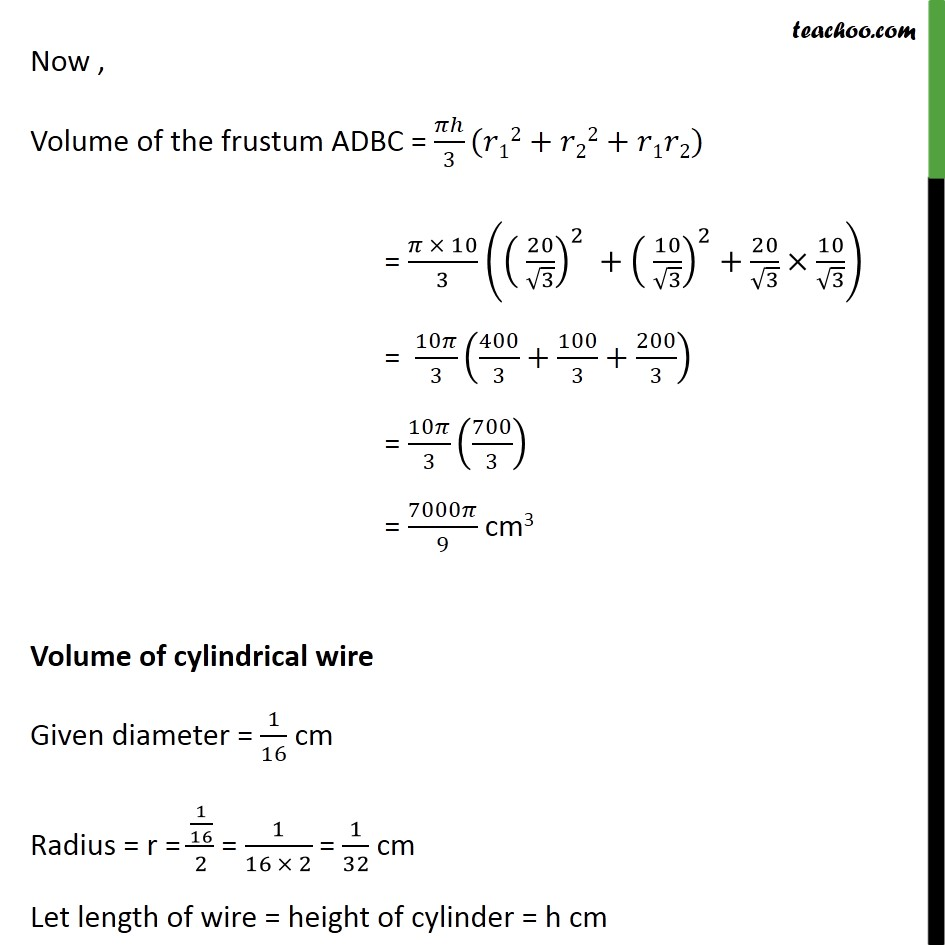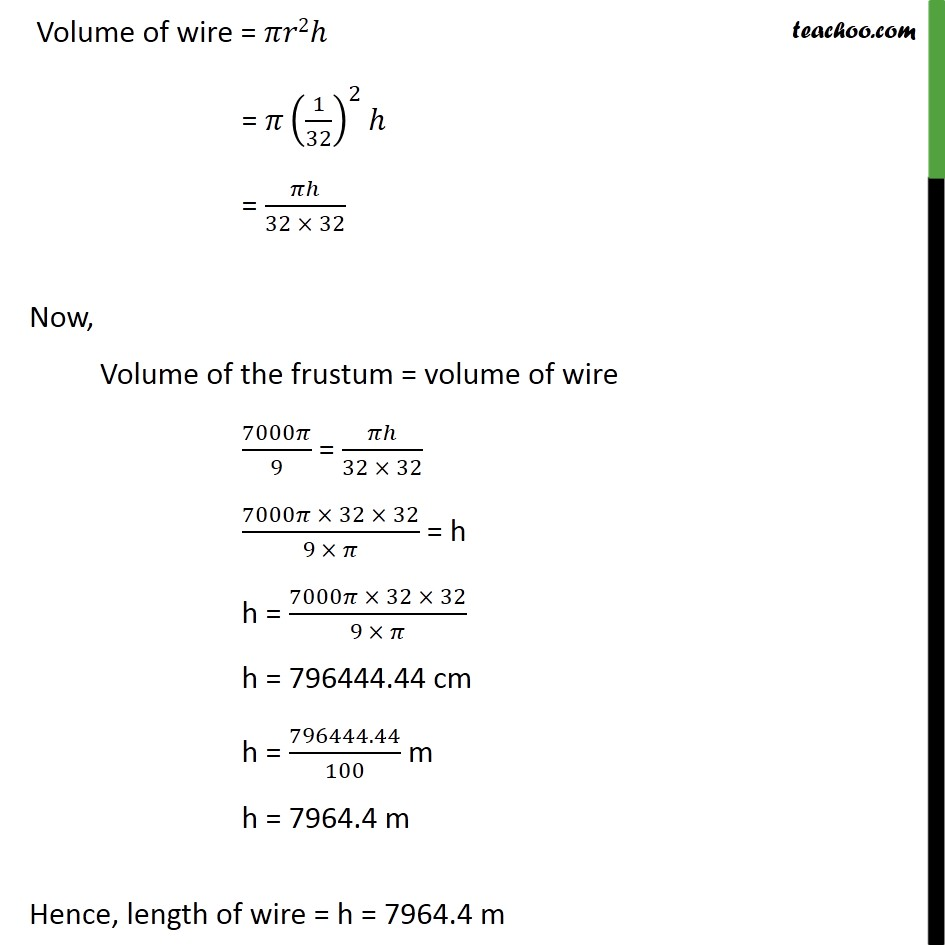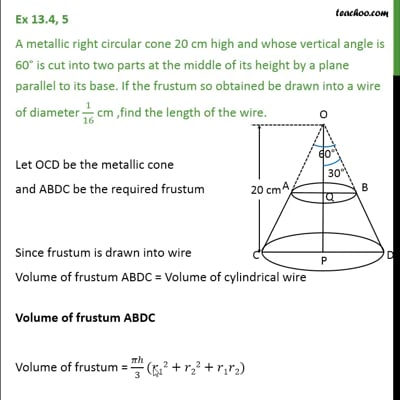This video is only available for Teachoo black users

Solve all your doubts with Teachoo Black (new monthly pack available now!)

### Transcript

Ex 13.4, 5 A metallic right circular cone 20 cm high and whose vertical angle is 60 is cut into two parts at the middle of its height by a plane parallel to its base. If the frustum so obtained be drawn into a wire of diameter 1/16 cm ,find the length of the wire. Let OCD be the metallic cone and ABDC be the required frustum Since frustum is drawn into wire Volume of frustum ABDC = Volume of cylindrical wire Volume of frustum ABDC Volume of frustum = /3( 12+ 22+ 1 2) Given OP = 20 cm Since cone is cut at the middle OQ = QP = 10 cm Also, QOB = 60 Height of frustum = h = QP = 10 cm We need to find r1 & r2 r1 = PD & r2 = QB Now , Volume of the frustum ADBC = /3( 12+ 22+ 1 2) = ( 10)/3 (( 20/ 3)^2 +( 10/ 3)^2+20/ 3 10/ 3) = 10 /3 (400/3+100/3+200/3) = 10 /3 (700/3) = 7000 /9 cm3 Volume of cylindrical wire Given diameter = 1/16 cm Radius = r = (1/16)/2 = 1/(16 2) = 1/32 cm Let length of wire = height of cylinder = h cm Volume of wire = 2 = (1/32)^2 = /(32 32) Now, Volume of the frustum = volume of wire 7000 /9 = /(32 32) (7000 32 32)/(9 ) = h h = (7000 32 32)/(9 ) h = 796444.44 cm h = 796444.44/100 m h = 7964.4 m Hence, length of wire = h = 7964.4 m